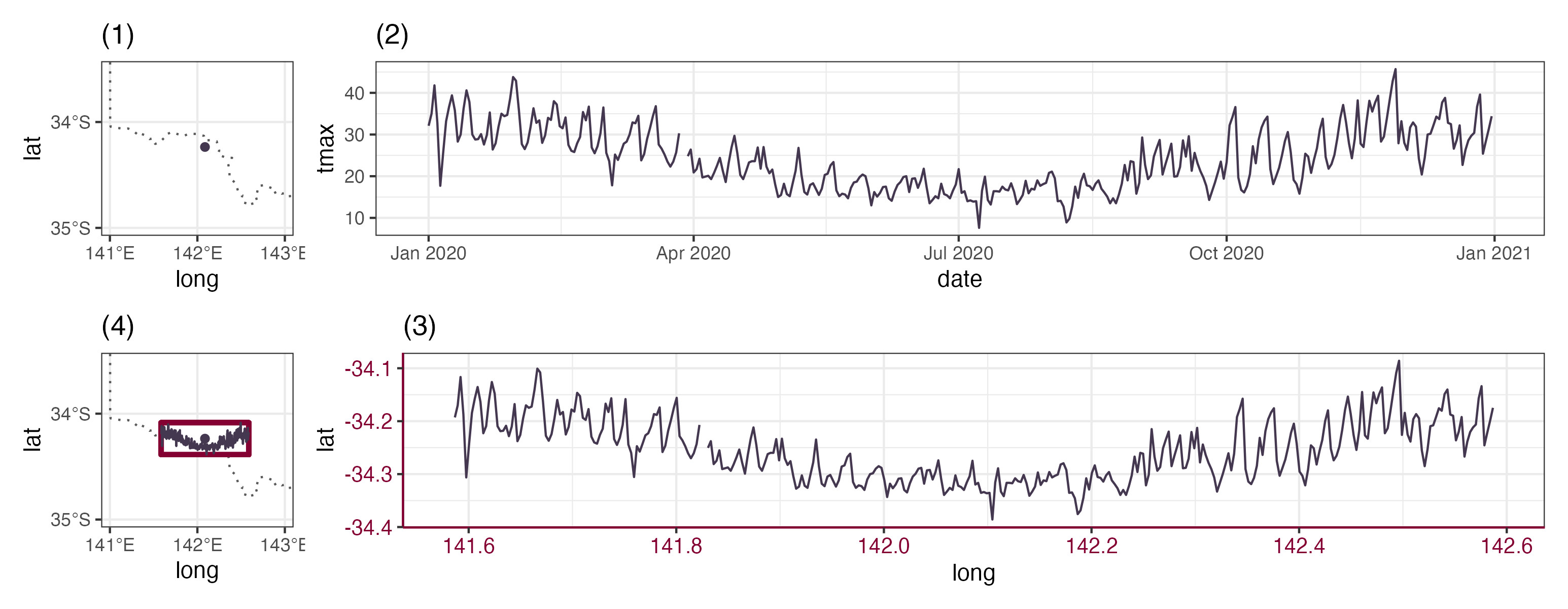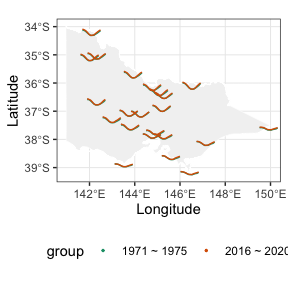# Making a glyph map

Sometimes, we wish to communicate spatial and temporal information collectively through visualisation. This can be done through making faceted maps across time, creating map animation, or in interactive graphics, constructing linking between maps and time series plot.

This vignette will introduce a type of spatio-temporal plot, glyph map, which displays spatial and temporal information in a single plot using linear algebra.

# What is a glyph map?

Glyph maps are initially proposed in Wickham et al. (2012) and the idea is to transform the temporal coordinates into the spatial coordinates so that time series plot can be displayed on the map. The following diagram illustrates how the transformation works:Subplot (1) and (2) show the location of a weather station and its associated maximum temperature in 2020. In (3), the same time series is transformed into the spatial coordinates with a defined height and width using linear algebra (Equation 1 in Wickham et al. (2012)). The transformed time series can then be placed on the map in (4). A polar transformation (Equation 2 in Wickham et al. (2012)) is also available with wrap the time series plot into a circle and it is useful to visualise seasonality.

The package GGally initially implement the glyph map. It uses glyphs() to calculate the axis transformation and then uses geom_polygon() to draw the map.

gly <- glyphs(data, x_major = ..., x_minor = ..., y_major = ..., y_minor = ..., ...)

# gx, gy, and gid are created within glyphs()
ggplot(gly, aes(gx, gy, group = gid)) +
geom_path()

Four variables are required to construct a glyph map: x_major, y_major, x_minor, and y_minor. The major axes are the coordinates used to create the map and in the illustration above x_major = long, y_major = lat. The minor axes are the x/y variable used to construct the time series plot(x_minor = date, y_minor = tmax in above).

# Glyph map in cubble

The cubble package implements the glyph map in a geom_glyph(), which perform the linear algebra internally as data transformation before the plot rendering. This allows you to use the conventionally aes() syntax within geom_glyph() to specify the four major/minor axes:

data |>
ggplot() +
geom_glyph(aes(x_major = ..., x_minor = ..., y_major = ..., y_minor = ...))

Reference line and box can be added by separate geoms (geom_glyph_box(), geom_glyph_line()) with the same aesthetics (x_major, x_minor, y_major, y_minor) and to avoid repetition, you may want specify them collectively in ggplot():

data |>
ggplot(aes(x_major = ..., x_minor = ..., y_major = ..., y_minor = ...)) +
geom_glyph_box() +
geom_glyph_line() +
geom_glyph()

If you want add additional layer to the plot, i.e. an undelying map, that does not use the four glyph map aesthetics, the argument inherit.aes = FALSE is handy:

data |>
ggplot(aes(x_major = ..., x_minor = ..., y_major = ..., y_minor = ...)) +
geom_sf(data = MAP_DATA, inherit.aes = FALSE)
geom_glyph_box() +
geom_glyph_line() +
geom_glyph()

Taking the output from the Get started page, we can visualise the different in avergae temperature curve between 1971 - 1975 and 2016 - 2020 using the glyph map:

vic_map <- ozmaps::abs_ste %>%  filter(NAME %in% c("Victoria"))
OUTPUT_FROM_GET_STARTED %>%
ggplot()  +
geom_sf(data = vic_map,
fill = "grey95", color = "white",
inherit.aes = FALSE) +
geom_glyph(
aes(x_major = longitude, y_major = latitude,
x_minor = month, y_minor = tmax,
group = interaction(id, group), color = group),
width = 0.8, height = 0.3) +
scale_color_brewer(palette = "Dark2") +
coord_sf(xlim = c(141, 150)) +
theme_bw() +
theme(legend.position = "bottom") +
labs(x = "Longitude", y = "Latitude")# Reference

Wickham, Hadley, Heike Hofmann, Charlotte Wickham, and Dianne Cook. 2012. “Glyph-Maps for Visually Exploring Temporal Patterns in Climate Data and Models.” Environmetrics 23 (5): 382–93. https://doi.org/10.1002/env.2152.